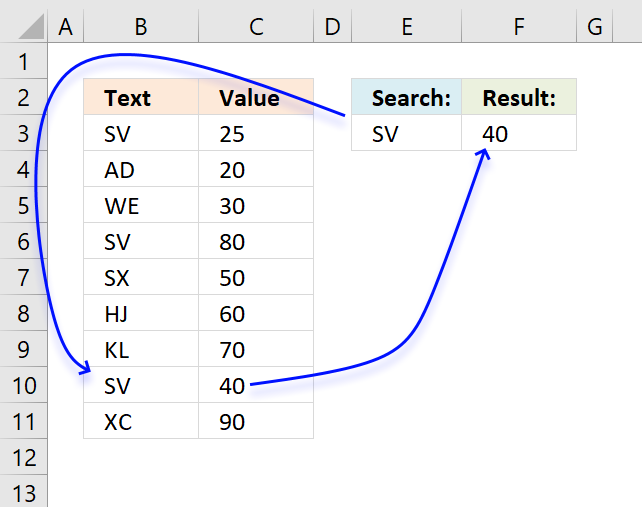Author: Oscar Cronquist Article last updated on November 16, 2020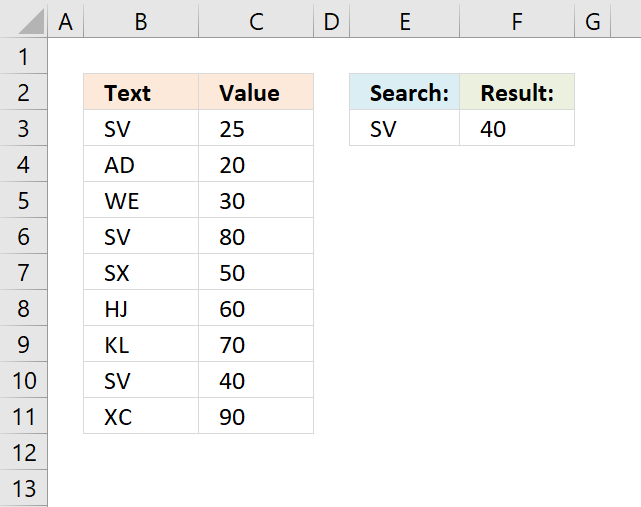This article demonstrates a formula that returns the last matching value based on a given condition. The above image shows the lookup value in cell E3 and the formula in cell F3.

The formula uses the condition in cell E3 to find the last matching value in cell range B3:B11 and returns the corresponding value on the same orw from cell range F3:F11.

I read an interesting blog post Find Last Item in Group With Index Match written by Debra Dalgleish. It is about finding the last matching value in a sorted list. It got me thinking how to find the last matching item in an unsorted list.

This picture shows random text strings in column B and values in column C. Cell E3 contains the search value and F3 contains an array formula that returns the last matching value in a list.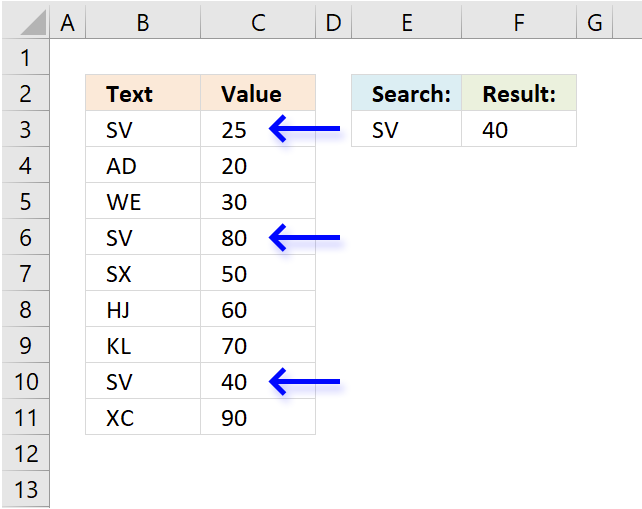Formula in cell F3:

=LOOKUP(2,1/(B3:B11=E3),C3:C11)

This formula is a regular formula if you are an Excel 365 subscriber, however, if you use an earlier Excel version you need to enter the formula as an array formula.

#### How to enter an array formula

1. Copy the above formula.
2. Double click with the left mouse button on cell F3.
3. Paste formula to cell.
4. Press and hold CTRL + SHIFT simultaneously.
5. Press Enter once.
6. Release all keys.

Find last matching value in an unsorted table

DonW asks: Ok, you've shown it for regular ranges....how about within tables. I have a table similar to: ID Name […]

### Explaining the formula in cell F3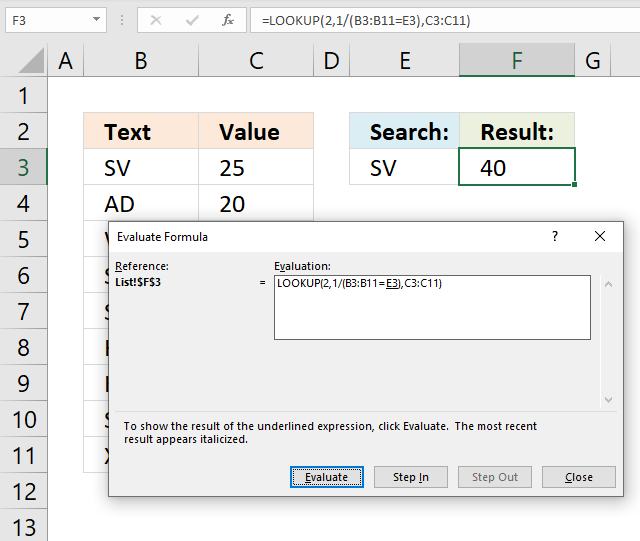The Evaluate Formula tool lets you see formula calculations in greater detail. Click with left mouse button on cell F3 to select it. Go to the "Formula" tab on the ribbon. Click the "Evaluate Formula" button and a dialog box appears, see above image.

You can now examine and troubleshoot the formula using the "Evaluate" button on the dialog box. It will, step by step, go through the calculation. The underlined expression is what is about to be evaluated and the italicized expression is the result of the most recent evaluation.

Keep clicking the "Evaluate" button to see all calculations. You will see the final result when all calculations are made, that value will match the value returned in cell F3. The "Close" button dismisses the dialog box when you are done evaluating.

#### Step 1 - Find matching values

The logical operators allow you to create a logical expression, they are : = < > and can also be combined.

= equal

> larger than

< smaller than

<> not equal to

=> larger than or equal to

=< smaller than or equal to

A logical expression returns a boolean value TRUE or FALSE. Note, they have numerical equivalents. TRUE anything but zero and FALSE = 0 (zero).

The following logical expression returns an array corresponding to cell range B3:B11.

B3:B11=E3

becomes

{"SV"; "AD"; "WE"; "SV"; "SX"; "HJ"; "KL"; "SV"; "XC"}="SV"

Arrays has delimiting characters, a ; (semicolon) means that the values are in a column. A , (comma) separates values in a row. An array may contain values from a cell range containing multiple rows and columns, this means that the array contains both ; and , to indicate their positions in the cell range.

returns

{TRUE; FALSE; FALSE; TRUE; FALSE; FALSE; FALSE; TRUE; FALSE}

TRUE means that the value is equal to the value in cell E3.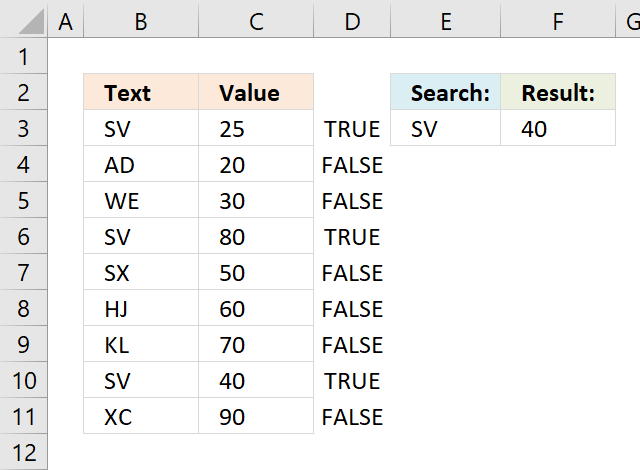#### Step 2 - Divide 1 with array

The LOOKUP function lets you find a value in a cell range and return a corresponding value on the same row, however, it also ignores error values and returns the last match which is surprising. I will show you how in step 3.

LOOKUP(lookup_value, lookup_vector, [result_vector])

The [result_vector] argument is optional. To create an error replacing the boolean value FALSE I simply divide 1 with the array.

1/(B3:B11=E3)

The parentheses allow you to control the order of operation meaning we want the formula to first calculate the logical expression and then divide 1 with the resulting array.

1/(B3:B11=E3)

becomes

1/{TRUE; FALSE; FALSE; TRUE; FALSE; FALSE; FALSE; TRUE; FALSE}

and returns

{1; #DIV/0!; #DIV/0!; 1; #DIV/0!; #DIV/0!; #DIV/0!; 1; #DIV/0!}

The #DIV/0! error is returned if you try to divide something with zero which is not possible.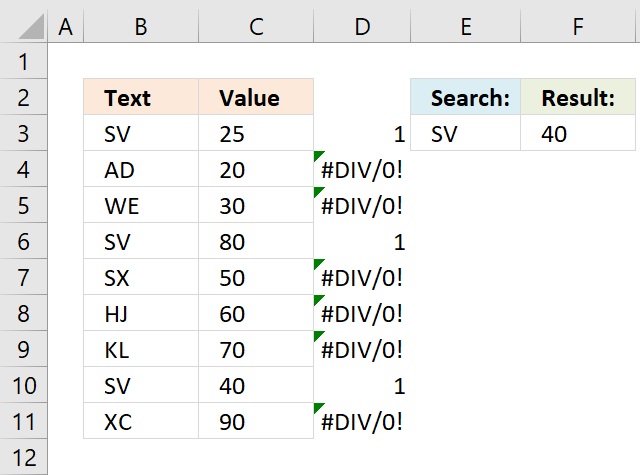The above image shows the array in cell range D3:D11, Excel shows a green triangle in the top left corner indicating that the value is an error value.

#### Step 3 - Find last value in array

The lookup_value must be larger than the values in the loop_vector and the values in the lookup_vector must be the same in order to get the last value that matches the lookup_value.

LOOKUP(lookup_value, lookup_vector, [result_vector])

LOOKUP(2,1/(B3:B11=E3),C3:C11)

becomes

LOOKUP(2,{1; #DIV/0!; #DIV/0!; 1; #DIV/0!; #DIV/0!; #DIV/0!; 1; #DIV/0!},C3:C11)

becomes

LOOKUP(2,{1; #DIV/0!; #DIV/0!; 1; #DIV/0!; #DIV/0!; #DIV/0!; 1; #DIV/0!},{25; 20; 30; 80; 50; 60; 70; 40; 90})

#### Step 4 - Return corresponding value

LOOKUP(2,1/(B3:B11=E3),C3:C11)

becomes

LOOKUP(2, {1;#DIV/0!;#DIV/0!;1; #DIV/0!;#DIV/0!; #DIV/0!;1;#DIV/0!}, {25; 20; 30; 80; 50; 60; 70; 40; 90})

and returns 40 in cell F3.

The last value matching the condition has the relative position eight in the array, the corresponding value in cell range C3:C11 is 40.